## Multiplying Fractions and Mixed Numbers

The steps involved in multiplying fractions and mixed numbers are similar, but an important first step is required when multiplying mixed numbers.

To multiply fractions, simply multiply the numerators; then multiply the denominators. Simplify to lowest terms if possible.

Multiply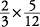.This answer had to be simplified because it wasn't in lowest terms.

When multiplying fractions, you can cancel (do early simplifying) first, which eliminates the need to simplify your answer later. To cancel, find a number that divides evenly into one numerator and one denominator. In Example , 2 will divide evenly into 2 in the numerator (it goes in one time) and 12 in the denominator (it goes in six times). Thus,Whole numbers can also be written as fractions, so the problemis worked by changing 3 to.

Multiply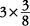.Multiply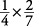.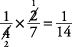Remember: You may cancel only when multiplying fractions.

To multiply mixed numbers, first change any mixed number to an improper fraction. Then multiply the numerators together and the denominators together, as shown in Example .

Multiply.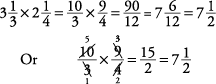Multiply.